# Algorithm Analysis Question 1 8My program finds all

• Slides: 45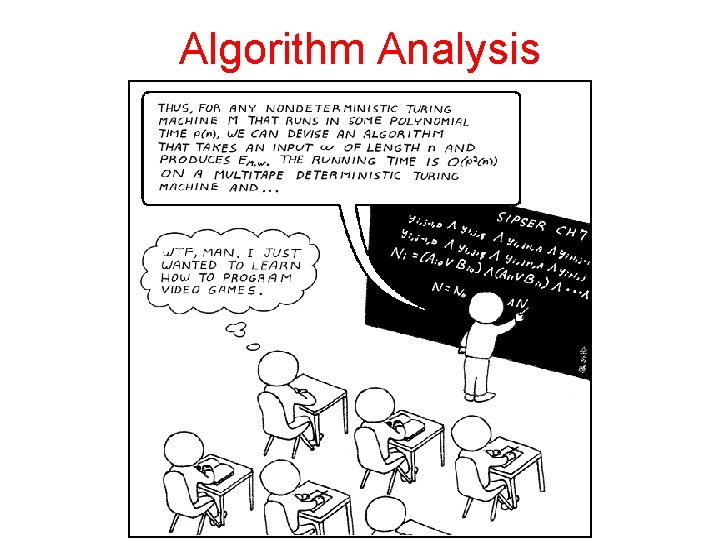Algorithm Analysis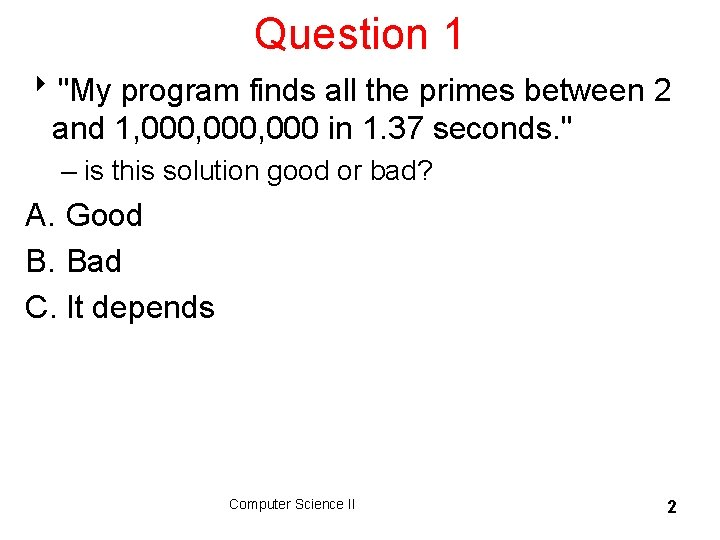Question 1 8"My program finds all the primes between 2 and 1, 000, 000 in 1. 37 seconds. " – is this solution good or bad? A. Good B. Bad C. It depends Computer Science II 2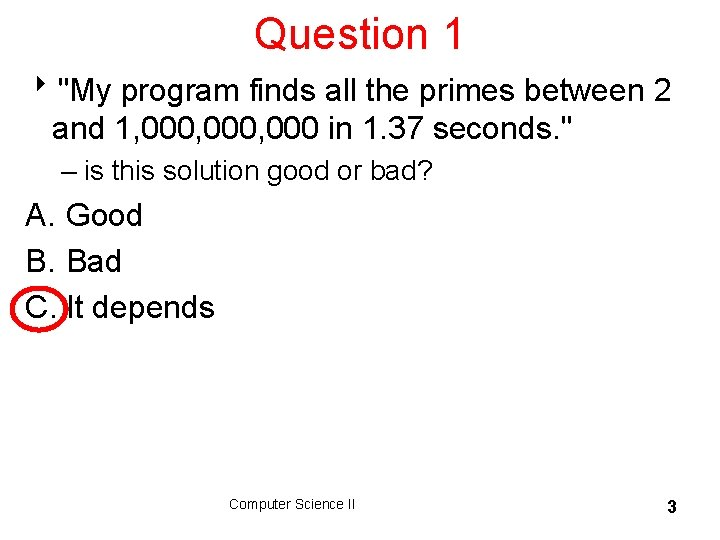Question 1 8"My program finds all the primes between 2 and 1, 000, 000 in 1. 37 seconds. " – is this solution good or bad? A. Good B. Bad C. It depends Computer Science II 3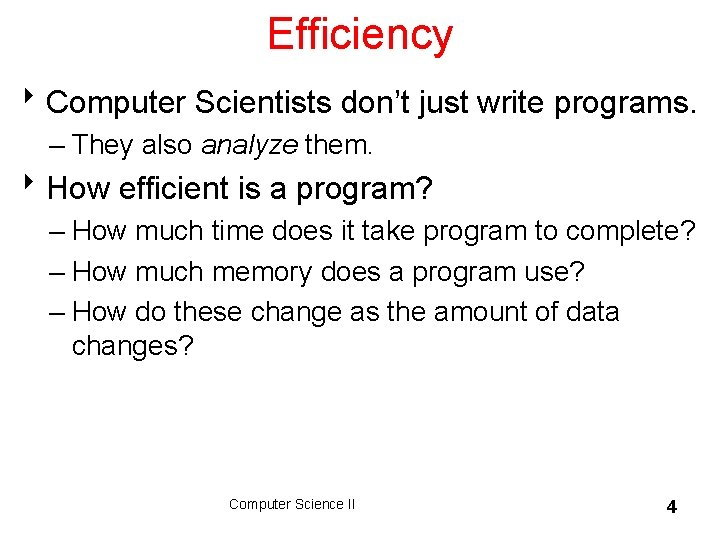Efficiency 8 Computer Scientists don’t just write programs. – They also analyze them. 8 How efficient is a program? – How much time does it take program to complete? – How much memory does a program use? – How do these change as the amount of data changes? Computer Science II 4Question 2 What is output by the following code? int total = 0; for(int i = 0; i < 13; i++) for(int j = 0; j < 11; j++) total += 2; System. out. println( total ); A. 24 B. 120 C. 143 D. 286 E. 338 Computer Science II 5Question 2 What is output by the following code? int total = 0; for(int i = 0; i < 13; i++) for(int j = 0; j < 11; j++) total += 2; System. out. println( total ); A. 24 B. 120 C. 143 D. 286 E. 338 Computer Science II 6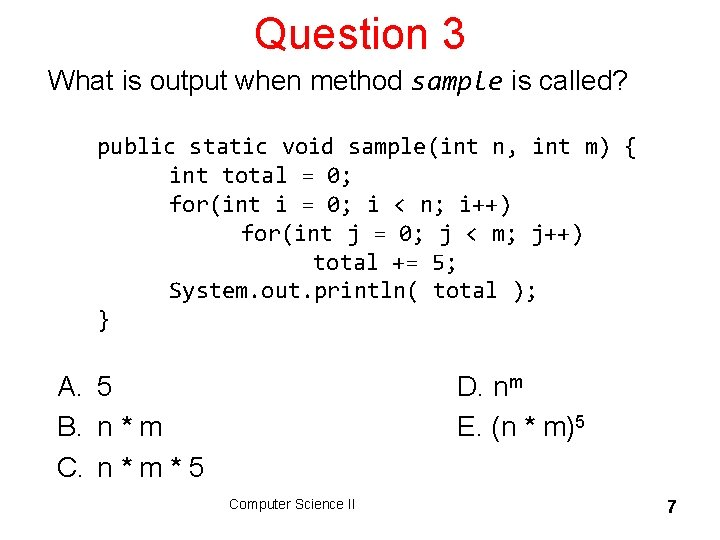Question 3 What is output when method sample is called? public static void sample(int n, int m) { int total = 0; for(int i = 0; i < n; i++) for(int j = 0; j < m; j++) total += 5; System. out. println( total ); } A. 5 B. n * m C. n * m * 5 D. nm E. (n * m)5 Computer Science II 7Question 3 What is output when method sample is called? public static void sample(int n, int m) { int total = 0; for(int i = 0; i < n; i++) for(int j = 0; j < m; j++) total += 5; System. out. println( total ); } A. 5 B. n * m C. n * m * 5 D. nm E. (n * m)5 Computer Science II 8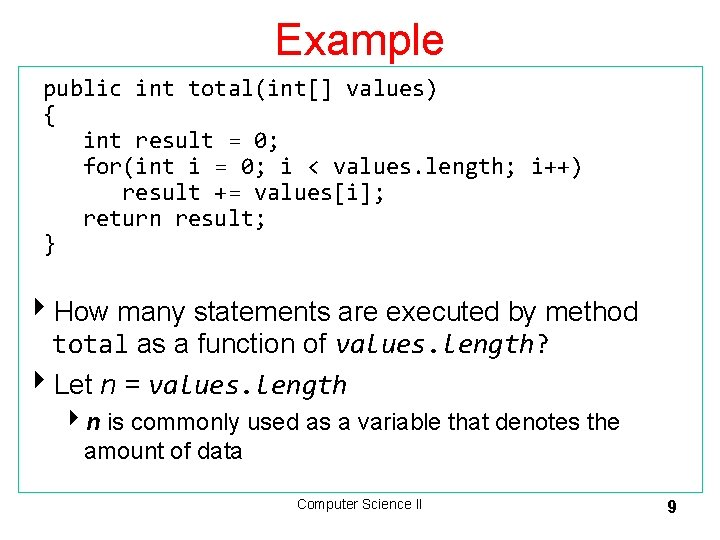Example public int total(int[] values) { int result = 0; for(int i = 0; i < values. length; i++) result += values[i]; return result; } 4 How many statements are executed by method total as a function of values. length? 4 Let n = values. length 4 n is commonly used as a variable that denotes the amount of data Computer Science II 9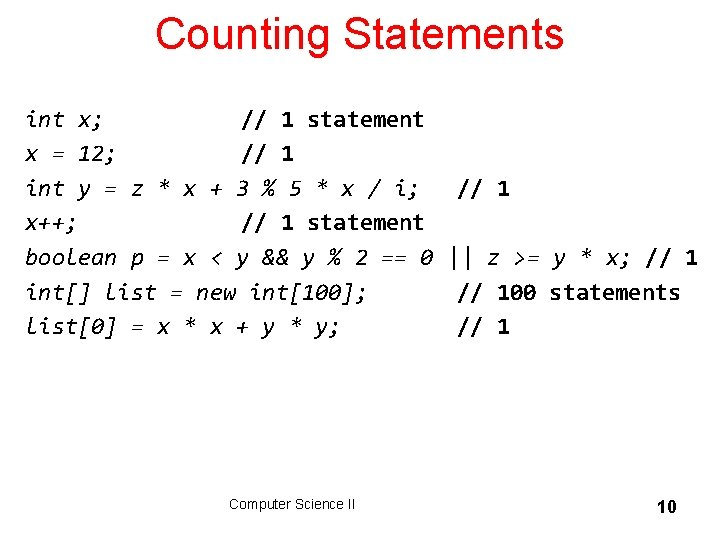Counting Statements int x; // 1 statement x = 12; // 1 int y = z * x + 3 % 5 * x / i; x++; // 1 statement boolean p = x < y && y % 2 == 0 int[] list = new int; list = x * x + y * y; Computer Science II // 1 || z >= y * x; // 100 statements // 1 10Counting Up Statements int result = 0; int i = 0; i < values. length; i++; result += values[i]; return total; T(n) = + 1 1 n+1 n n 1 _ 3 n + 4 T(n) is the number of executable statements in method total as function of values. length = n Computer Science II 11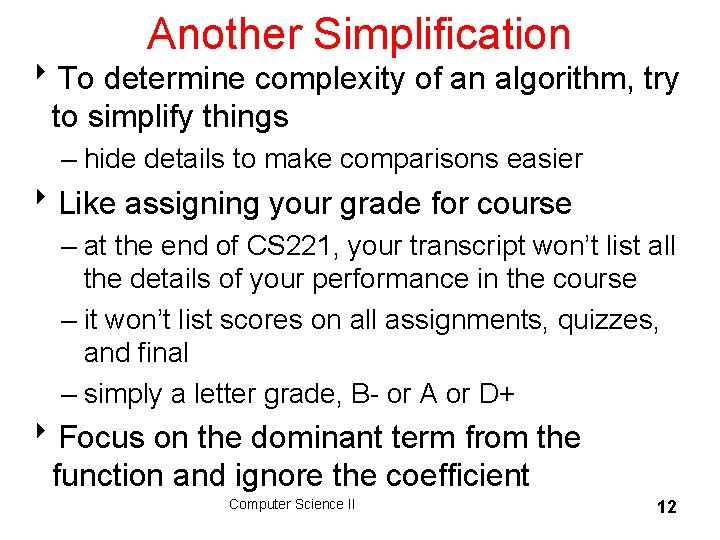Another Simplification 8 To determine complexity of an algorithm, try to simplify things – hide details to make comparisons easier 8 Like assigning your grade for course – at the end of CS 221, your transcript won’t list all the details of your performance in the course – it won’t list scores on all assignments, quizzes, and final – simply a letter grade, B- or A or D+ 8 Focus on the dominant term from the function and ignore the coefficient Computer Science II 12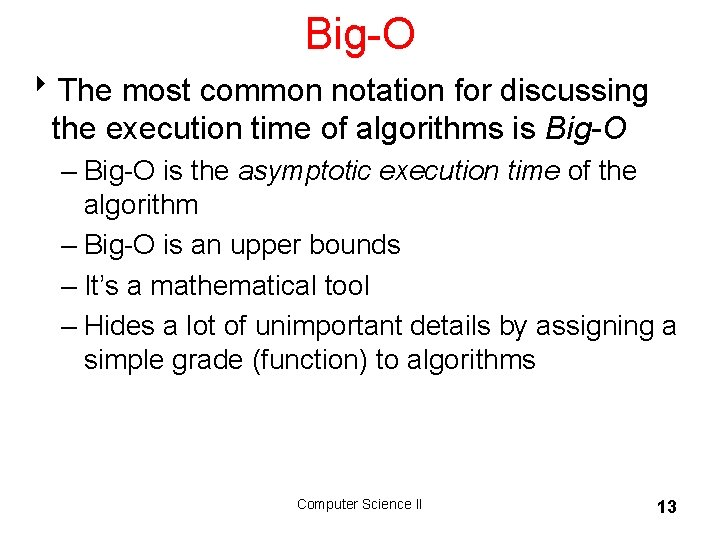Big-O 8 The most common notation for discussing the execution time of algorithms is Big-O – Big-O is the asymptotic execution time of the algorithm – Big-O is an upper bounds – It’s a mathematical tool – Hides a lot of unimportant details by assigning a simple grade (function) to algorithms Computer Science II 13Formal Definition of Big-O 8 T(n) is in O( g(n) ) if there are positive constants c and n 0 such that T(n) < c*g(n) for all n > n 0 – n is the size of the data set the algorithm works on – T(n) is a function that characterizes the actual running time of the algorithm – g(n) is a function that characterizes an upper bounds on T(n). It is an upper limit on the running time of the algorithm. – c and n 0 are constants Computer Science II 14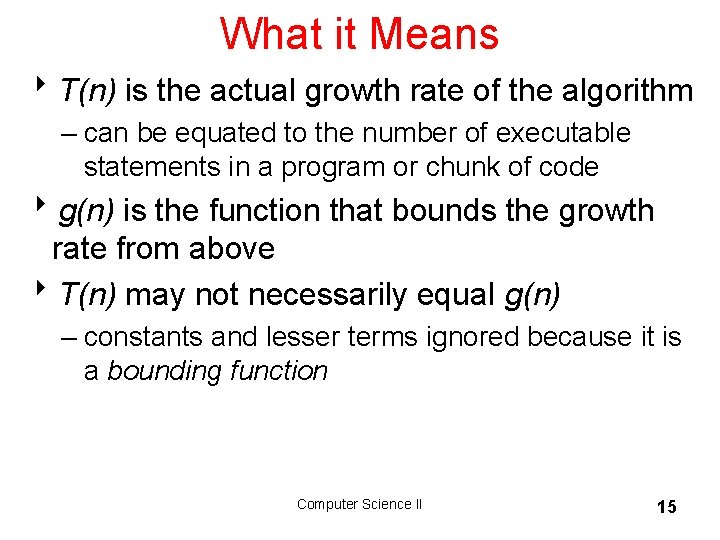What it Means 8 T(n) is the actual growth rate of the algorithm – can be equated to the number of executable statements in a program or chunk of code 8 g(n) is the function that bounds the growth rate from above 8 T(n) may not necessarily equal g(n) – constants and lesser terms ignored because it is a bounding function Computer Science II 15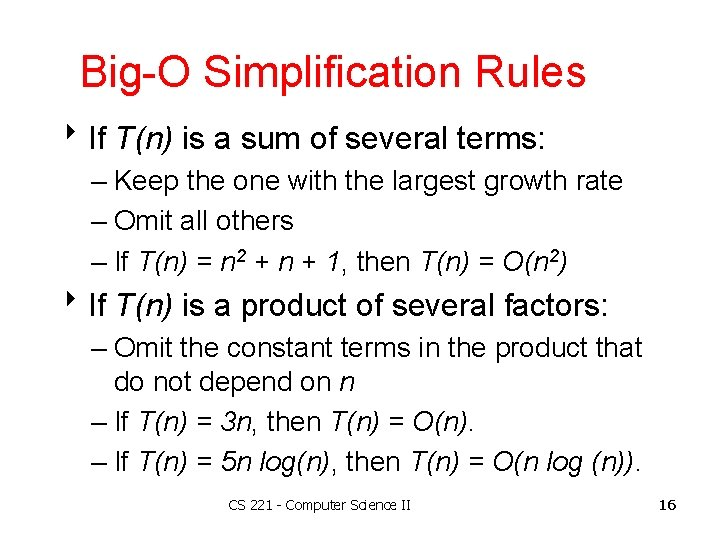Big-O Simplification Rules 8 If T(n) is a sum of several terms: – Keep the one with the largest growth rate – Omit all others – If T(n) = n 2 + n + 1, then T(n) = O(n 2) 8 If T(n) is a product of several factors: – Omit the constant terms in the product that do not depend on n – If T(n) = 3 n, then T(n) = O(n). – If T(n) = 5 n log(n), then T(n) = O(n log (n)). CS 221 - Computer Science II 16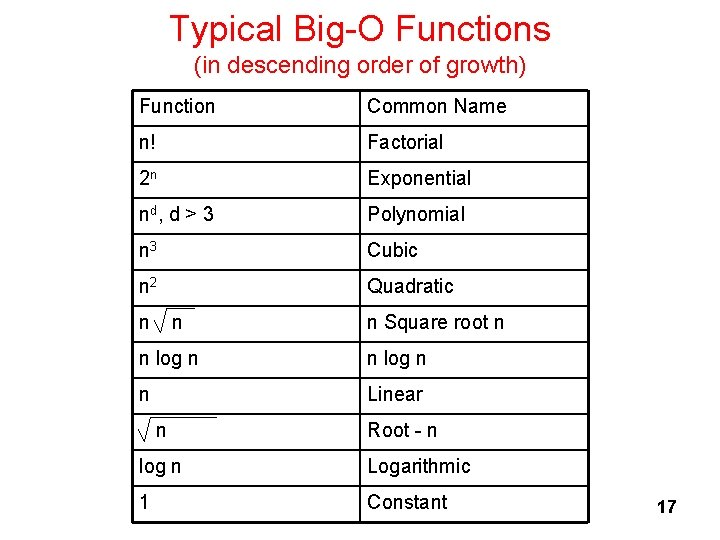Typical Big-O Functions (in descending order of growth) Function Common Name n! Factorial 2 n Exponential nd , d > 3 Polynomial n 3 Cubic n 2 Quadratic n n n Square root n n log n n Linear n Root - n log n Logarithmic 1 Constant 17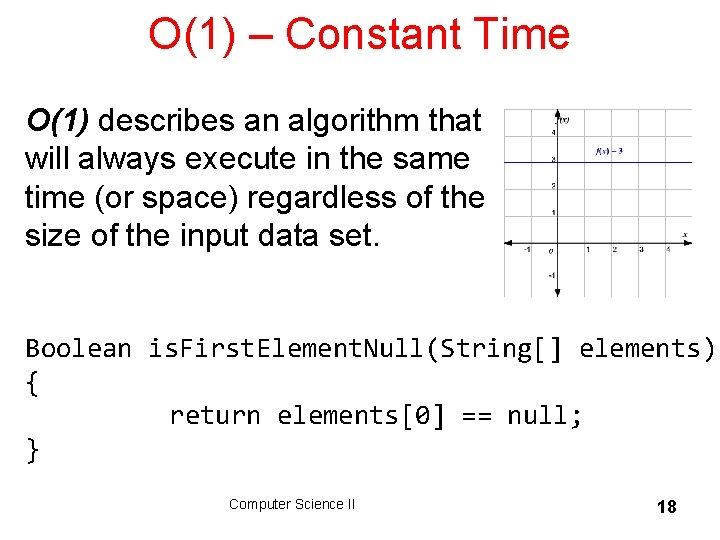O(1) – Constant Time O(1) describes an algorithm that will always execute in the same time (or space) regardless of the size of the input data set. Boolean is. First. Element. Null(String[] elements) { return elements == null; } Computer Science II 18O(log n) – Logarithmic Time Iterative halving of data sets • doubling the size of the input data set has little effect on its growth. • after a single iteration, the data set will be halved and therefore on a par with an input data set half the size. Algorithms like Binary Search, which run in logarithmic time, are extremely efficient when dealing with large data sets. Computer Science II 19Binary Search int binary_search(int A[], int key, int min, int max) { // test if array is empty if (max < min) // set is empty, so return value showing not found return KEY_NOT_FOUND; else { // calculate midpoint to cut set in half int mid = midpoint(min, max); // three-way comparison if (A[mid] > key) // key is in lower subset return binary_search(A, key, min, mid - 1); else if (A[mid] < key) // key is in upper subset return binary_search(A, key, mid + 1, max); else // key has been found return mid; } } 20O(n) – Linear Time O(n) describes an algorithm whose performance will grow linearly and in direct proportion to the size of the input data set. Boolean contains. Value(String[] elements, string value, int size) { for(int i = 0; i < size; i++) { if (element == value) return true; } return false; } Computer Science II 21O(n 2) – Quadratic Time O(n 2) represents an algorithm whose performance is directly proportional to the square of the size of the input data set. – Usually algorithms that involve nested iterations over the data set. – Deeper nested iterations will result in O(n 3), O(n 4) etc. Computer Science II 22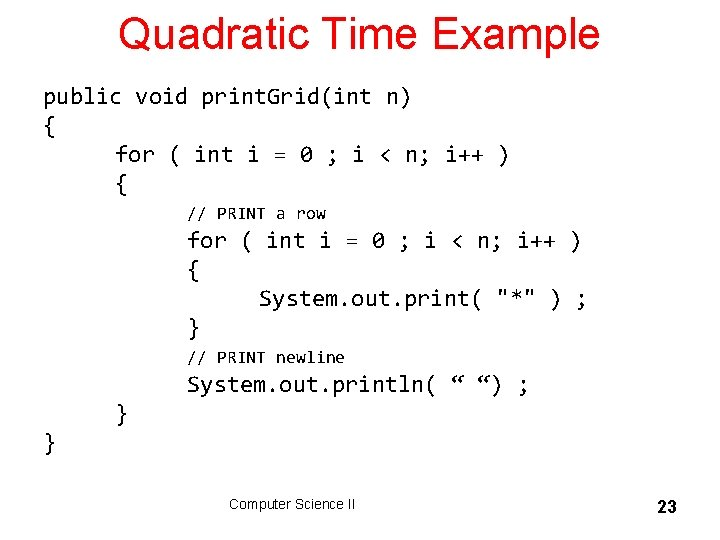Quadratic Time Example public void print. Grid(int n) { for ( int i = 0 ; i < n; i++ ) { // PRINT a row for ( int i = 0 ; i < n; i++ ) { System. out. print( "*" ) ; } // PRINT newline System. out. println( “ “) ; } } Computer Science II 23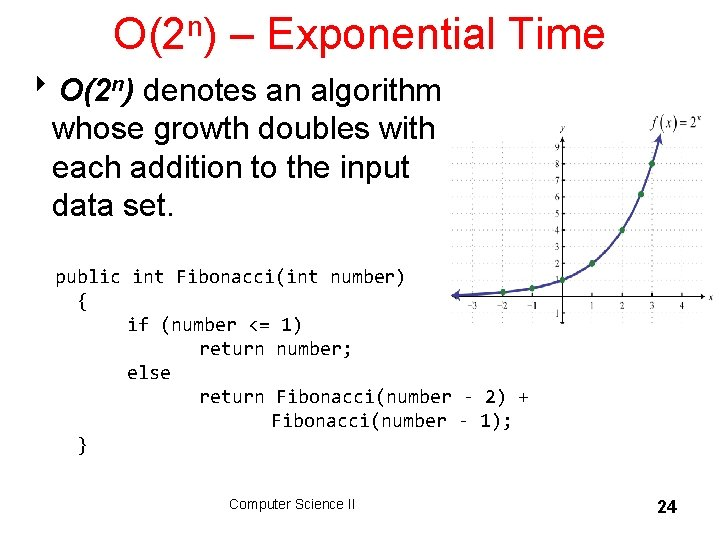O(2 n) – Exponential Time 8 O(2 n) denotes an algorithm whose growth doubles with each addition to the input data set. public int Fibonacci(int number) { if (number <= 1) return number; else return Fibonacci(number - 2) + Fibonacci(number - 1); } Computer Science II 24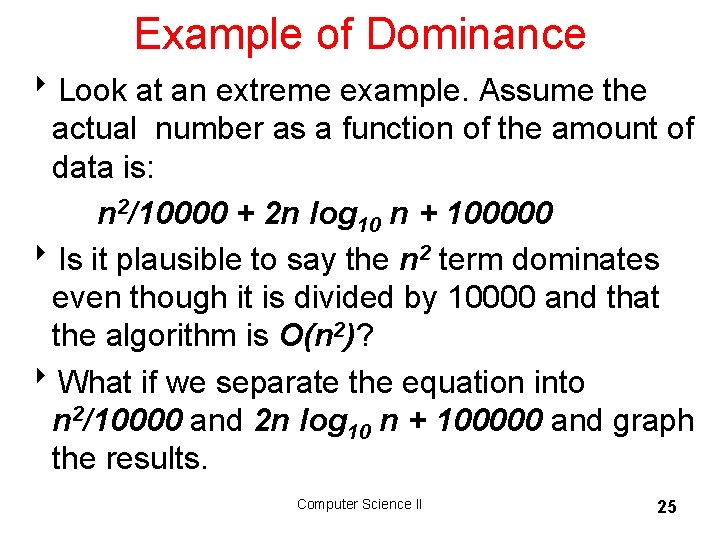Example of Dominance 8 Look at an extreme example. Assume the actual number as a function of the amount of data is: n 2/10000 + 2 n log 10 n + 100000 8 Is it plausible to say the n 2 term dominates even though it is divided by 10000 and that the algorithm is O(n 2)? 8 What if we separate the equation into n 2/10000 and 2 n log 10 n + 100000 and graph the results. Computer Science II 25Summing Execution Times red line is 2 n log 10 n + 100000 blue line is n 2/10000 8 For large values of n the n 2 term dominates so the algorithm is O(n 2) 8 When does it make sense to use a computer? Computer Science II 26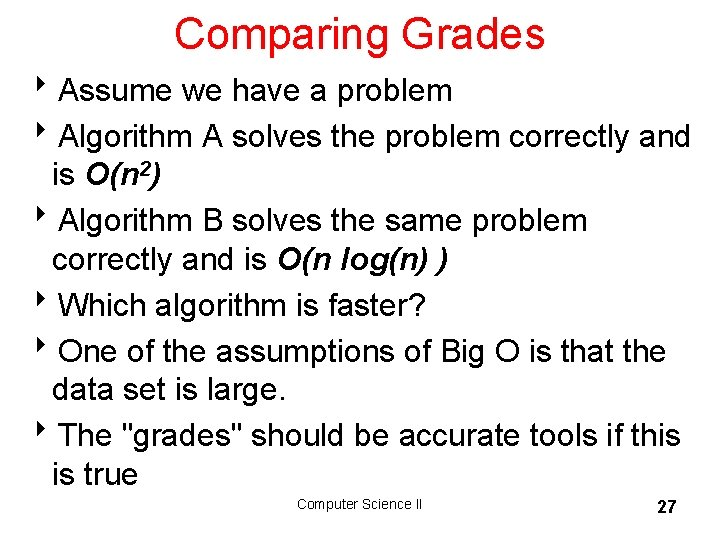Comparing Grades 8 Assume we have a problem 8 Algorithm A solves the problem correctly and is O(n 2) 8 Algorithm B solves the same problem correctly and is O(n log(n) ) 8 Which algorithm is faster? 8 One of the assumptions of Big O is that the data set is large. 8 The "grades" should be accurate tools if this is true Computer Science II 27Running Times 8 Assume n = 100, 000 and processor speed is 1, 000, 000 operations per second Function Running Time 2 n 3. 2 x 1030086 years n 4 3171 years n 3 11. 6 days n 2 10 seconds n n 0. 032 seconds n log n 0. 0017 seconds n 0. 0001 seconds n 3. 2 x 10 -7 seconds log n 1. 2 x 10 -8 seconds Computer Science II 28Just Count Loops, Right? // assume mat is a 2 d array of booleans // assume mat is square with n rows, // and n columns int num. Things = 0; for(int r = row - 1; r <= row + 1; r++) for(int c = col - 1; c <= col + 1; c++) if( mat[r][c] ) num. Things++; What is the order of the above code? A. O(1) B. O(n) C. O(n 2) Computer Science II D. O(n 3) E. O(n 1/2) 29It is Not Just Counting Loops // Example from previous slide rewritten as follows: int if( if( if( num. Things = 0; mat[r-1][c-1] ) num. Things++; mat[r-1][c+1] ) num. Things++; mat[r][c-1] ) num. Things++; mat[r][c+1] ) num. Things++; mat[r+1][c-1] ) num. Things++; mat[r+1][c+1] ) num. Things++; Computer Science II 30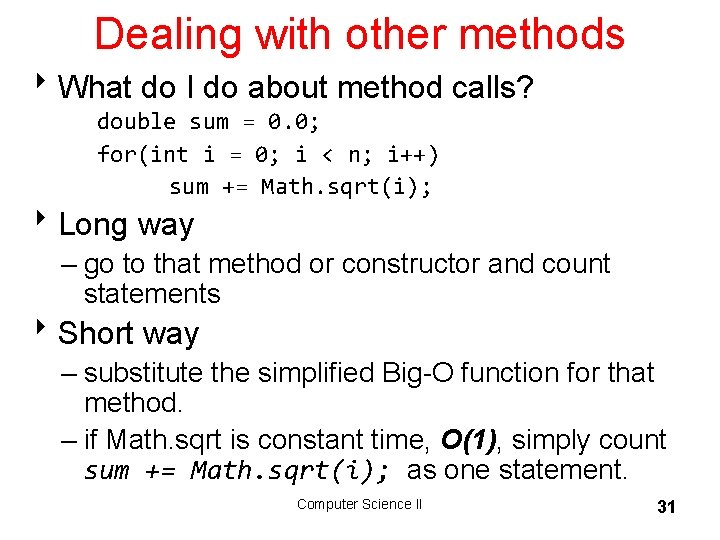Dealing with other methods 8 What do I do about method calls? double sum = 0. 0; for(int i = 0; i < n; i++) sum += Math. sqrt(i); 8 Long way – go to that method or constructor and count statements 8 Short way – substitute the simplified Big-O function for that method. – if Math. sqrt is constant time, O(1), simply count sum += Math. sqrt(i); as one statement. Computer Science II 31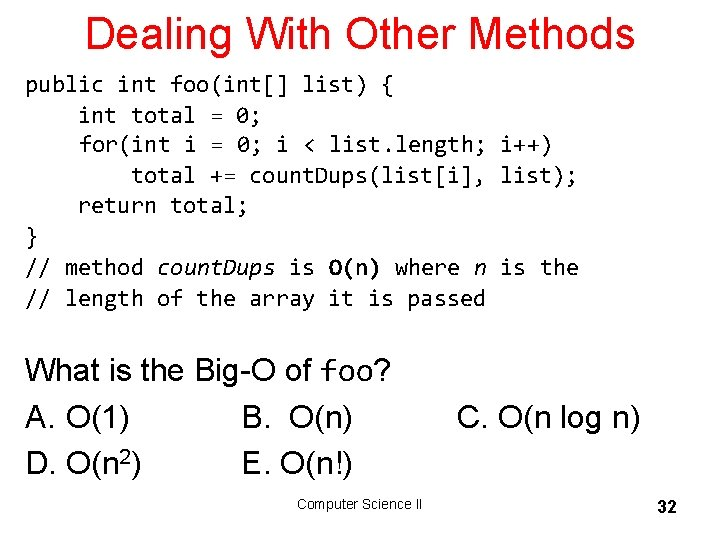Dealing With Other Methods public int foo(int[] list) { int total = 0; for(int i = 0; i < list. length; i++) total += count. Dups(list[i], list); return total; } // method count. Dups is O(n) where n is the // length of the array it is passed What is the Big-O of foo? A. O(1) B. O(n) D. O(n 2) E. O(n!) Computer Science II C. O(n log n) 32Dealing With Other Methods public int foo(int[] list) { int total = 0; for(int i = 0; i < list. length; i++) total += count. Dups(list[i], list); return total; } // method count. Dups is O(n) where n is the // length of the array it is passed What is the Big-O of foo? A. O(1) B. O(n) D. O(n 2) E. O(n!) Computer Science II C. O(n log n) 33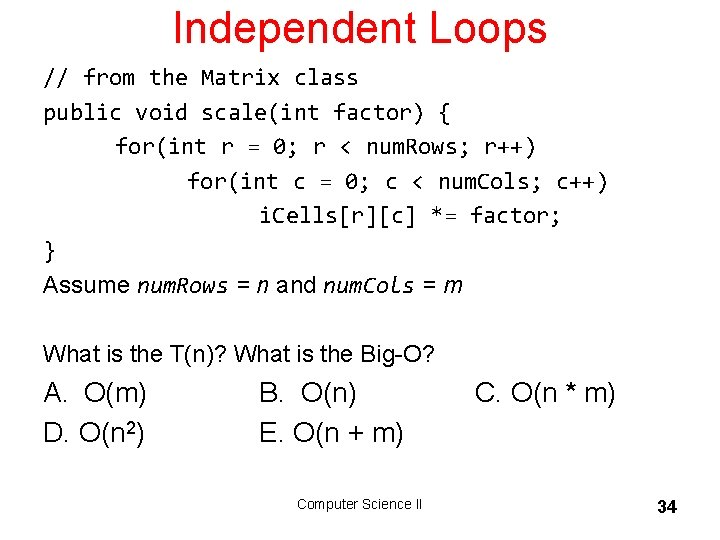Independent Loops // from the Matrix class public void scale(int factor) { for(int r = 0; r < num. Rows; r++) for(int c = 0; c < num. Cols; c++) i. Cells[r][c] *= factor; } Assume num. Rows = n and num. Cols = m What is the T(n)? What is the Big-O? A. O(m) D. O(n 2) B. O(n) E. O(n + m) Computer Science II C. O(n * m) 34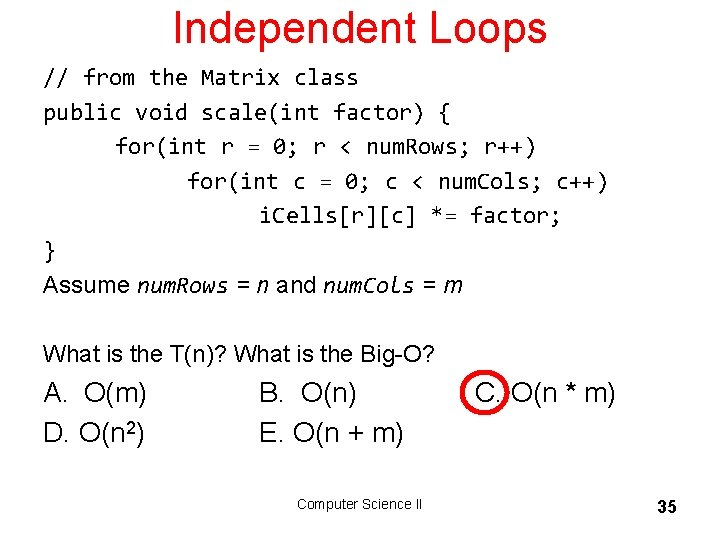Independent Loops // from the Matrix class public void scale(int factor) { for(int r = 0; r < num. Rows; r++) for(int c = 0; c < num. Cols; c++) i. Cells[r][c] *= factor; } Assume num. Rows = n and num. Cols = m What is the T(n)? What is the Big-O? A. O(m) D. O(n 2) B. O(n) E. O(n + m) Computer Science II C. O(n * m) 35Why Use Big-O? 8 As we build data structures, Big-O is the tool we will use to decide under what conditions one data structure is better than another 8 Think about performance when there is a lot of data. – "It worked so well with small data sets. . . " – Joel Spolsky, Schlemiel the painter's Algorithm 8 Lots of trade offs – some data structures good for certain types of problems, bad for other types – often able to trade SPACE for TIME. – Faster solution that uses more space – Slower solution that uses less space Computer Science II 36Big-O Space 8 Big-O could be used to specify how much space is needed for a particular algorithm – in other words how many variables are needed 8 Often there is a time – space tradeoff – can often take less time if willing to use more memory – can often use less memory if willing to take longer – truly beautiful solutions take less time and space The biggest difference between time and space is that you can't reuse time. - Merrick Furst Computer Science II 37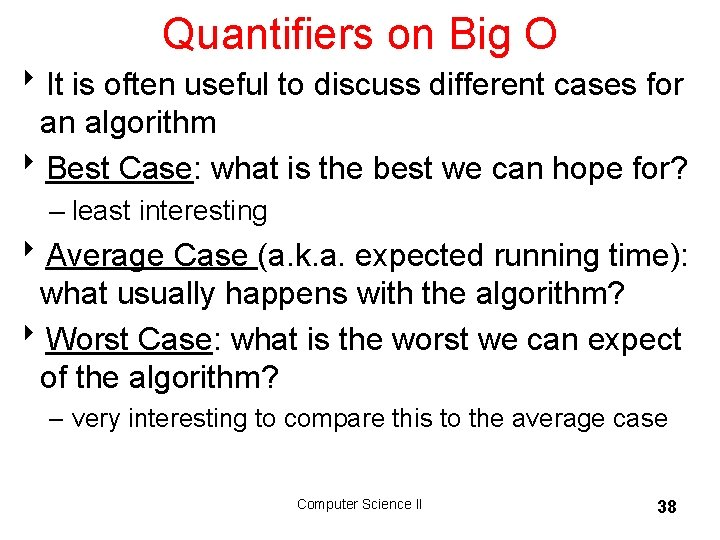Quantifiers on Big O 8 It is often useful to discuss different cases for an algorithm 8 Best Case: what is the best we can hope for? – least interesting 8 Average Case (a. k. a. expected running time): what usually happens with the algorithm? 8 Worst Case: what is the worst we can expect of the algorithm? – very interesting to compare this to the average case Computer Science II 38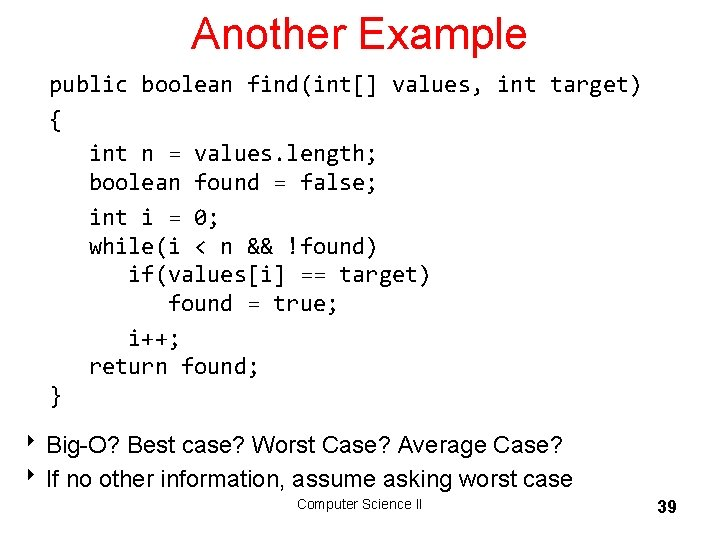Another Example public boolean find(int[] values, int target) { int n = values. length; boolean found = false; int i = 0; while(i < n && !found) if(values[i] == target) found = true; i++; return found; } 8 Big-O? Best case? Worst Case? Average Case? 8 If no other information, assume asking worst case Computer Science II 39Computer Science II 40Showing O(n) is Correct 8 Recall the formal definition of Big O – T(n) is O( g(n) ) if there are positive constants c and n 0 such that T(n) < c*g(n) when n > n 0 8 Recall method total, T(n) = 3 n + 4 – show method total is O(n). – g(n) is n 8 We need to choose constants c and n 0 8 how about c = 4, n 0 = 5 ? Computer Science II 41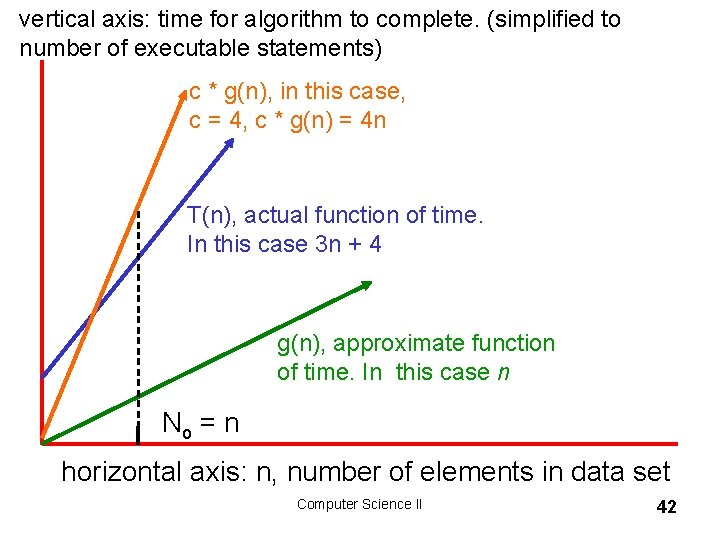vertical axis: time for algorithm to complete. (simplified to number of executable statements) c * g(n), in this case, c = 4, c * g(n) = 4 n T(n), actual function of time. In this case 3 n + 4 g(n), approximate function of time. In this case n No = n horizontal axis: n, number of elements in data set Computer Science II 42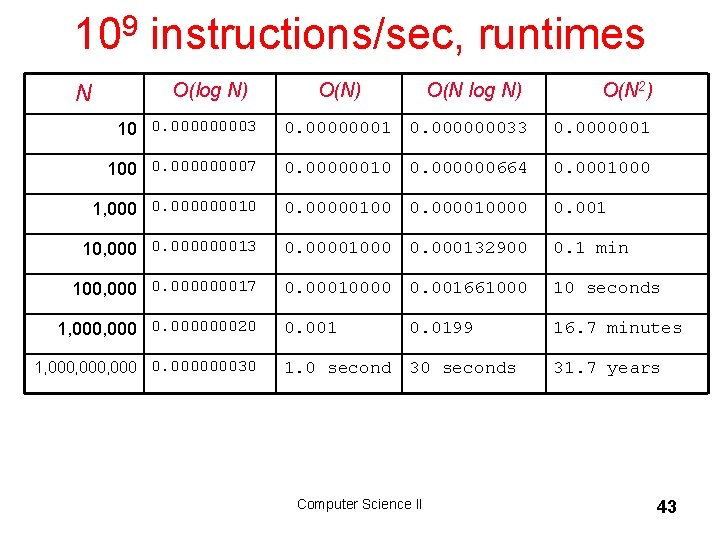109 instructions/sec, runtimes N O(log N) O(N 2) 10 0. 00003 0. 00000001 0. 000000033 0. 0000001 100 0. 00007 0. 00000010 0. 000000664 0. 0001000 1, 000 0. 000000010 0. 00000100 0. 000010000 0. 001 10, 000 0. 000000013 0. 00001000 0. 000132900 0. 1 min 100, 000 0. 000000017 0. 00010000 0. 001661000 10 seconds 0. 001 16. 7 minutes 1, 000 0. 000000020 1, 000, 000 0. 000000030 0. 0199 1. 0 second 30 seconds Computer Science II 31. 7 years 43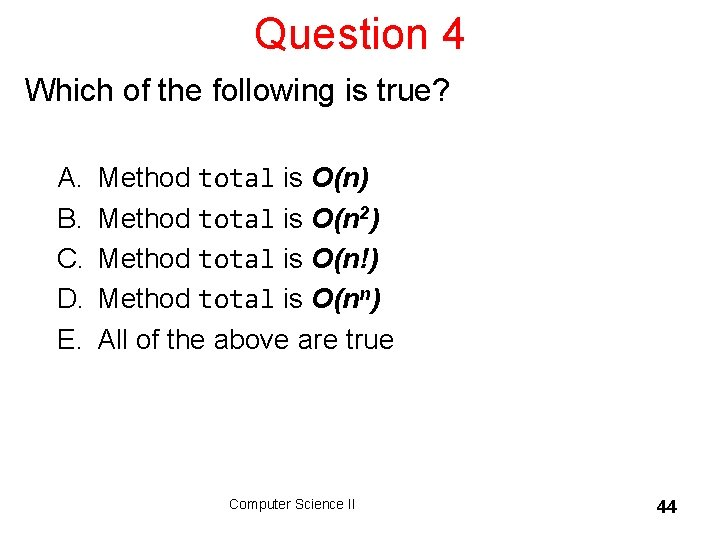Question 4 Which of the following is true? A. B. C. D. E. Method total is O(n) Method total is O(n 2) Method total is O(n!) Method total is O(nn) All of the above are true Computer Science II 44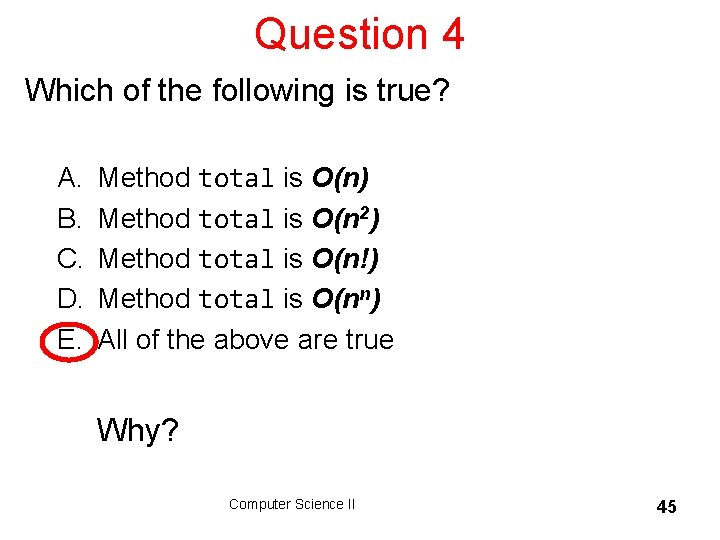Question 4 Which of the following is true? A. B. C. D. E. Method total is O(n) Method total is O(n 2) Method total is O(n!) Method total is O(nn) All of the above are true Why? Computer Science II 45